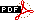«Информационные технологии и системы 2015» 39-я междисциплинарная школа-конференция 7 – 11 сентября, Олимпийская деревня, Сочи, Россия ISBN: 978-5-901158-28-9Русский | English

Подписаться
на новости

Организаторы:

 Среда, 9 сентября14:30 - 15:30Флагман 3 Сессия: Оптимизация и транспортНауки о данных Председатель: д.ф.-м.н. Спокойный Владимир Григорьевич

Дерендяев А.Б.
Оценка скорости трафика по данным мобильного оператораАннотация: A method for estimating the dynamics of urban traffic velocity with the data from the mobile operator is considered. A model allowing to calculate the approximate speed on road sections and to estimate congestion of these sections is proposed. The method is implemented on the GIS platform GeoTime 3. Experimental re-sults for the road network of Moscow city and Moscow region are discussed.

Бедринцев А.А., Чепыжов В.В.
Описание пространства дизайна с помощью экстремальных эллипсоидовАннотация: Problems of data set description and outliers detection are solved by constructing optimal ellipsoid. Optimization problems are formulated as convex programming problems using linear matrix inequalities. Proposed method is compared with similar known before methods in terms of two criteria: volume of ellipsoid and the number of points in train data set that lie beyond the ellipsoid.

Двуреченский П.Е., Гасников А.В.
Стохастический промежуточный градиентный метод: выпуклый и сильно выпуклый случайАннотация: In this paper we introduce new methods for convex optimization problems with inexact stochastic oracle. First method is an extension of the intermediate gradient method proposed by Devolder, Glineur and Nesterov for problems with inexact oracle. Our new method can be applied to the problems with composite structure, stochastic inexact oracle and allows using non-Euclidean setup. We prove estimates for mean rate of convergence and probabilities of large deviations from this rate. Also we introduce two modifications of this method for strongly convex problems. For the first modification we prove mean rate of convergence estimates and for the second we prove estimates for large deviations from the mean rate of convergence. All the rates give the complexity estimates for proposed methods which up to multiplicative constant coincide with lower complexity bound for the considered class of convex composite optimization problems with stochastic inexact oracle.